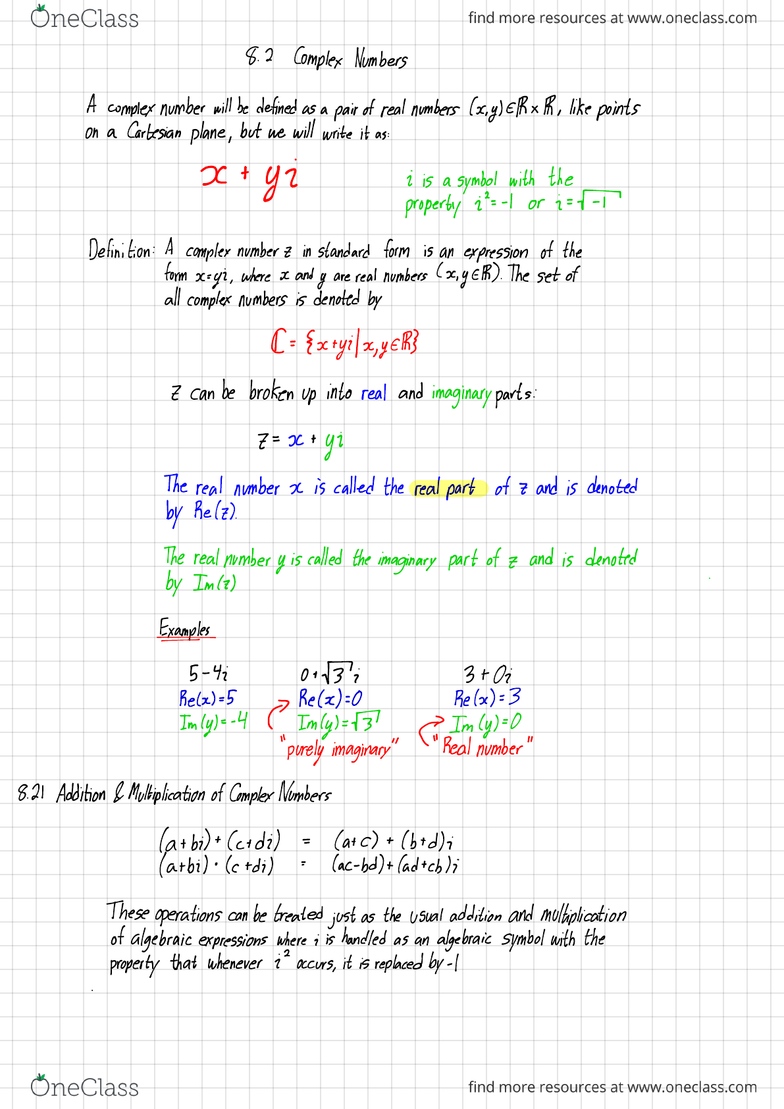Textbook Notes (270,000)
CA (160,000)
McMaster (10,000)
MATH (400)
Chapter 8.2

# MATH 1C03 Chapter Notes - Chapter 8.2: Local Access And Transport Area, Prope, Uver River

Department
Mathematics
Course Code
MATH 1C03
Professor
Miroslav Lovric
Chapter
8.2

Page:
of 48.2 Complex Numbers
Acomplex number will be defined as apair of real numbers (x.gl EIRXR ,like points
on aCartesian plane ,but we will write it as :
XtYI iis asymbol with the
property it -Ior i=F
Definition :Acomplex number zin standard form is an expression of the
form x=yi ,where xand yare real numbers (x,yER ).The set of
all complex numbers is denoted by
E- {xtyilx,YER}
Zcan be broken up into real and imaginary parts :
z= x+Yi
The real number xis called the real part of 7and is denoted
by Retz).
The real number yis called the imaginary part of zand is denoted
by Imla ).
Ex¥÷
-ti o+Mi 3+02 .
Rebel =5 Re (x):O Relx )=3
Imlyt -4 PImly )=D >In (y)=O
"
purely imaginary
"󱚩
Real number "
8.21 Addition &Multiplication of Complex Numbers
(at bi)+(ctdi )=(at
c) +(bid );
(at bi ).(ctdi )=(ac -bd)+(adtcbli
These operations can be treated just as the usual
of algebraic expressions where iis handled as an algebraic Symbol with the
property
that whenever is occurs ,it is replaced by -1
.
Example
÷4;)+(3ti )(5+4
;) .(3ti )(2-3 ;)•#.
)(
1+42=(5+3)+1++1
.)i =5.3+4.31+5 .li+4i2 =;-ftp.2 =I+2itj2
=8+5 ;=15+17 ;
-4=1+2;
-1=11+17
;=3zti =2;
Consider these operations ontwocomplexnumberswithzeroimainary parts
(a+0i)+( Ctoi )=(a)+0 ;real addition
(attn ).¢t0i )=actoi real multiplication
This means that all real numbers lie on the complex
plane ,and .therefore
thesetof real numbers (Bhisasvbsetotthe complex plane (¢)
Wehavenow achieved our objective of
extending the
number system to include the
Square roots of all negative numbers
.
Example
÷
-z=4tiandw=-3i2i be complex numbers.Find
Dztw ii )z -wNoteipowersofi
z.,w=H+i)H -3+2 ;) z.wftti )-(-3+21)=7 .ilifn :O (modt)
=1+3 ;=7 -jiifn=1(mod4 )
iiiew nine
in ¥iHnIYem
:b:p
z2w=( tti.pt -3+2.)w4=( -3+2 ;)t
H6t8itiYC3t2il =-34+41-31312 ;) '- 4×-3512.5+41-3 )fzjH2D"
=t5+8i)f3t2 ;) -
-81-216 ;+216in
-963+14.4=-45-24
;
-138+167=81-216
;i96i+l6
=-61+6 ;=-119-120 ;
Proposition
-
Let Z,w,
tEE and let a=xtgi and w=u +vi
where
,
x,y,v.v ER .Then :
i)
,?
=wif and only if x=o and y=v
Two
complex numbers are equal if and only if their real parts
are equal &their imaginary parts are equal
Proof:The complex numbers z=x+yi and w=u+vi represent
the ordered
pair of real numbers (x,y)and (v.v )
respectively .These two ordered pairs are equal it
and only if their first elements are equal and their
Second elements are equal
ii)(z + w)it =z+(wit )(associatively of addition
).
iii)z+w= wtz (com mutativity of addition )
iv )0=0+0 ithen 0+7=2-1
existence of azero )
v)The number -z =-x-Yi EE is such that ¥+1 -z)=O (existence of negatives)
vi)(z.w).t =zfw .tl lasso ciativity of multiplication)
vii Z.w=W.z(com mutativity of multiplication
.)
viii)1=1+0 ;is such that I.z=z (existence of aunit )
Dz .(wit )=z.W+zt (distributive law)
Proof:All these
parts of the proposition can be proved by
calculating each expression directly.
ix )If zto ,the element
Z
''
=zt =x-yi=
-y2
is the inverse of zand satisfies zz
''=I (existence of inverses )
Proof :The
complex number z=x+yi is zero if and only if x=o and
y=O.Hence it Zto ,then either xor yis nonzero ,and it follows
that oity '>0
.Therefore
I7÷z=x÷y -Itta
(
×j+Yg÷D( xtgi )=x2txg=y =1
x2+y 2
Hence
the complex number ,xjyy÷ is the inverse of xiyi